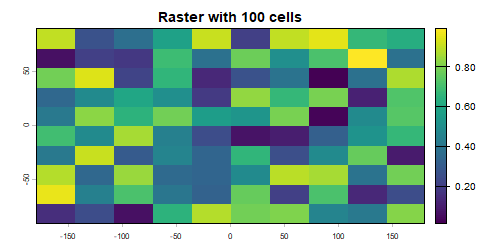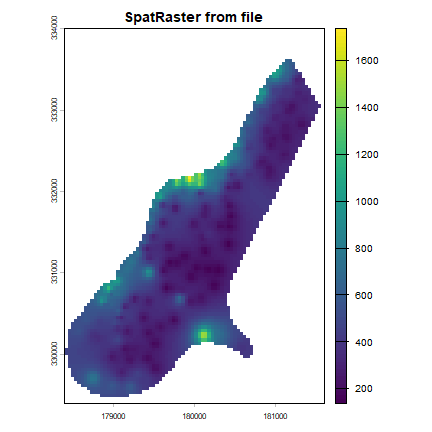# Creating Raster* objects¶

A RasterLayer can easily be created from scratch using the function raster. The default settings will create a global raster data structure with a longitude/latitude coordinate reference system and 1 by 1 degree cells. You can change these settings by providing additional arguments such as xmin, nrow, ncol, and/or crs, to the function. You can also change these parameters after creating the object. If you set the projection, this is only to properly define it, not to change it. To transform a RasterLayer to another coordinate reference system (projection) you can use the function ** projectRaster**.

Here is an example of creating and changing a RasterLayer object ‘r’ from scratch.

library(raster)
# RasterLayer with the default parameters
x <- raster()
x
## class      : RasterLayer
## dimensions : 180, 360, 64800  (nrow, ncol, ncell)
## resolution : 1, 1  (x, y)
## extent     : -180, 180, -90, 90  (xmin, xmax, ymin, ymax)
## crs        : +proj=longlat +datum=WGS84 +ellps=WGS84 +towgs84=0,0,0
# With other parameters
x <- raster(ncol=36, nrow=18, xmn=-1000, xmx=1000, ymn=-100, ymx=900)
# that can be changed
res(x)
##  55.55556 55.55556
# change resolution
res(x) <- 100
res(x)
##  100 100
ncol(x)
##  20
# change the numer of columns (affects resolution)
ncol(x) <- 18
ncol(x)
##  18
res(x)
##  111.1111 100.0000
# set the coordinate reference system (CRS) (define the projection)
projection(x) <- "+proj=utm +zone=48 +datum=WGS84"
x
## class      : RasterLayer
## dimensions : 10, 18, 180  (nrow, ncol, ncell)
## resolution : 111.1111, 100  (x, y)
## extent     : -1000, 1000, -100, 900  (xmin, xmax, ymin, ymax)
## crs        : +proj=utm +zone=48 +datum=WGS84 +ellps=WGS84 +towgs84=0,0,0


The object x created in the example above only consist of a “skeleton”, that is, we have defined the number of rows and columns, and where the raster is located in geographic space, but there are no cell-values associated with it. Setting and accessing values is illustrated below.

r <- raster(ncol=10, nrow=10)
ncell(r)
##  100
hasValues(r)
##  FALSE
# use the 'values' function
# e.g.,
values(r) <- 1:ncell(r)
# or
set.seed(0)
values(r) <- runif(ncell(r))
hasValues(r)
##  TRUE
inMemory(r)
##  TRUE
values(r)[1:10]
##   0.8966972 0.2655087 0.3721239 0.5728534 0.9082078 0.2016819 0.8983897
##   0.9446753 0.6607978 0.6291140
plot(r, main='Raster with 100 cells')In some cases, for example when you change the number of columns or rows, you will lose the values associated with the RasterLayer if there were any (or the link to a file if there was one). The same applies, in most cases, if you change the resolution directly (as this can affect the number of rows or columns). Values are not lost when changing the extent as this change adjusts the resolution, but does not change the number of rows or columns.

hasValues(r)
##  TRUE
res(r)
##  36 18
dim(r)
##  10 10  1
xmax(r)
##  180
# change the maximum x coordinate of the extent (bounding box) of the RasterLayer
xmax(r) <- 0
hasValues(r)
##  TRUE
res(r)
##  18 18
dim(r)
##  10 10  1
ncol(r) <- 6
hasValues(r)
##  FALSE
res(r)
##  30 18
dim(r)
##  10  6  1
xmax(r)
##  0


The function raster also allows you to create a RasterLayer from another object, including another RasterLayer, RasterStack and RasterBrick , as well as from a SpatialPixels* and SpatialGrid* object (defined in the sp package), an Extent object, a matrix, an ‘im’ object (SpatStat), and ‘asc’ and ‘kasc’ objects (adehabitat).

It is more common, however, to create a RasterLayer object from a file. The raster package can use raster files in several formats, including some ‘natively’ supported formats and other formats via the rgdal package. Supported formats for reading include GeoTIFF, ESRI, ENVI, and ERDAS. Most formats supported for reading can also be written to. Here is an example using the ‘Meuse’ dataset (taken from the sp package), using a file in the native ‘raster-file’ format:

# get the name of an example file installed with the package
# do not use this construction of your own files
filename <- system.file("external/test.grd", package="raster")
filename
##  "C:/soft/R/R-3.6.0/library/raster/external/test.grd"
r <- raster(filename)
filename(r)
##  "C:\\soft\\R\\R-3.6.0\\library\\raster\\external\\test.grd"
hasValues(r)
##  TRUE
inMemory(r)
##  FALSE
plot(r, main='RasterLayer from file')Multi-layer objects can be created in memory (from RasterLayer objects) or from files.

# create three identical RasterLayer objects
r1 <- r2 <- r3 <- raster(nrow=10, ncol=10)
# Assign random cell values
values(r1) <- runif(ncell(r1))
values(r2) <- runif(ncell(r2))
values(r3) <- runif(ncell(r3))
# combine three RasterLayer objects into a RasterStack
s <- stack(r1, r2, r3)
s
## class      : RasterStack
## dimensions : 10, 10, 100, 3  (nrow, ncol, ncell, nlayers)
## resolution : 36, 18  (x, y)
## extent     : -180, 180, -90, 90  (xmin, xmax, ymin, ymax)
## crs        : +proj=longlat +datum=WGS84 +ellps=WGS84 +towgs84=0,0,0
## names      :    layer.1,    layer.2,    layer.3
## min values : 0.01307758, 0.02778712, 0.06380247
## max values :  0.9926841,  0.9815635,  0.9960774
nlayers(s)
##  3
# combine three RasterLayer objects into a RasterBrick
b1 <- brick(r1, r2, r3)
# equivalent to:
b2 <- brick(s)
# create a RasterBrick  from file
filename <- system.file("external/rlogo.grd", package="raster")
filename
##  "C:/soft/R/R-3.6.0/library/raster/external/rlogo.grd"
b <- brick(filename)
b
## class      : RasterBrick
## dimensions : 77, 101, 7777, 3  (nrow, ncol, ncell, nlayers)
## resolution : 1, 1  (x, y)
## extent     : 0, 101, 0, 77  (xmin, xmax, ymin, ymax)
## crs        : +proj=merc +datum=WGS84 +ellps=WGS84 +towgs84=0,0,0
## source     : C:/soft/R/R-3.6.0/library/raster/external/rlogo.grd
## names      : red, green, blue
## min values :   0,     0,    0
## max values : 255,   255,  255
nlayers(b)
##  3
# extract a single RasterLayer
r <- raster(b, layer=2)
# equivalent to creating it from disk
r <- raster(filename, band=2)